# What time is it?

What time is it, when there is four times less time left until midnight than the time that has elapsed since noon? What time is it, when one-fifth of the hours that have passed since midnight is equal to one-third of the hours that are missing by 12 o'clock?

Result

a = 14:24 hh:mmb = 7:30 hh:mm### Step-by-step explanation:

a-12 = (24-a)/4

5a = 72

a = 72/5 = 14.4

Our simple equation calculator calculates it.

b/5 = (12-b)/3

8b = 60

b = 15/2 = 7.5

Our simple equation calculator calculates it.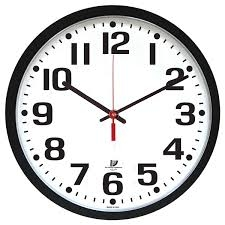Did you find an error or inaccuracy? Feel free to write us. Thank you!Tips to related online calculators
Need help to calculate sum, simplify or multiply fractions? Try our fraction calculator.
Do you have a linear equation or system of equations and looking for its solution? Or do you have a quadratic equation?
Do you want to convert time units like minutes to seconds?

## Related math problems and questions:

• MidnightHow many hours are, if the time that elapsed since 8:00 is 2/5 of the time that will past till midnight?
• Clock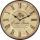What time is now if the time elapsed afternoon is 2/10 of time that elapses before midnight?
• Clock hands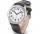The hands-on clock shows the time 12 hours and 2 minutes. Calculate the size of an acute angle between clock hands three hours later.
• Tourist routeHow long is the tourist route when tourists crossed four-sevenths of the way on foot, crossed the bus twice less than on foot, and passed the last 14 kilometers by boat?
• Grandmother's clocks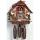Grandmother's clock is late every hour by half a minute. Grandmother set the clock exactly at 8.00 am. How many hours will show after 24 hours?
• Fraction equationEx over four is equal to two and one-fifth times ex plus three over ten. What is ex>
• Delayed clockMichael put a new battery into his watch at midnight. However, they are 5 seconds late each minute. How many hours does the watch show in 24 hours?
• PlayingHow long have we trained on the pitch when we know that the warm-up took 10 minutes, we trained passes for one-third of the time and we played football half the time?
• SchoolLess than 500 pupils attend school. When it is sorted into pairs, one pupil remains. Similarly, when sorted into 3, 4, 5 and 6 members team one remains. Sorted to seven members teams, no left behind. How many pupils are attending this school?
• Fifth of the numberA fifth of the number is by 24 less than that number. What is the number?
• SymmetryEva loves symmetry in shapes and numbers. Yesterday she invented a completely new kind of symmetry - divisible symmetry. She wrote all five-digit numbers with different digits with the following property: the first digit is divisible by 1, the second by 2
• Strawberries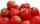Father collects strawberries himself in 4 hours, son in 14 hours. How long will it take them along when dad comes to help his son collect strawberries after 3 hours?
• Who IWho I am? My third is 2 less than my half.
• Tailor master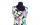There are less than 50m of textile in the tailoring workshop. When cutting on a blouse (consumption 1.5m), no textile is left. When using a cloth (consumption of 3.2m), no textile is left. How many meters of textile are in a tailor's workshop?
• Stamps and albumsMichail has in three albums stored 170 stamps. In the first album, there are 14 more than in the second and in the second is by 1/5 less than in the third. How many stamps are in the first album?
• Four pupilsFour pupils divided \$ 1485 so that the second received 50% less than the first, the third 1/2 less than a fourth, and fourth \$ 154 less than the first. How much money had each of them?
• Two numbersThe sum of two numbers is 1. Identify this two numbers if you know that the half of first is equal to the third of second number.